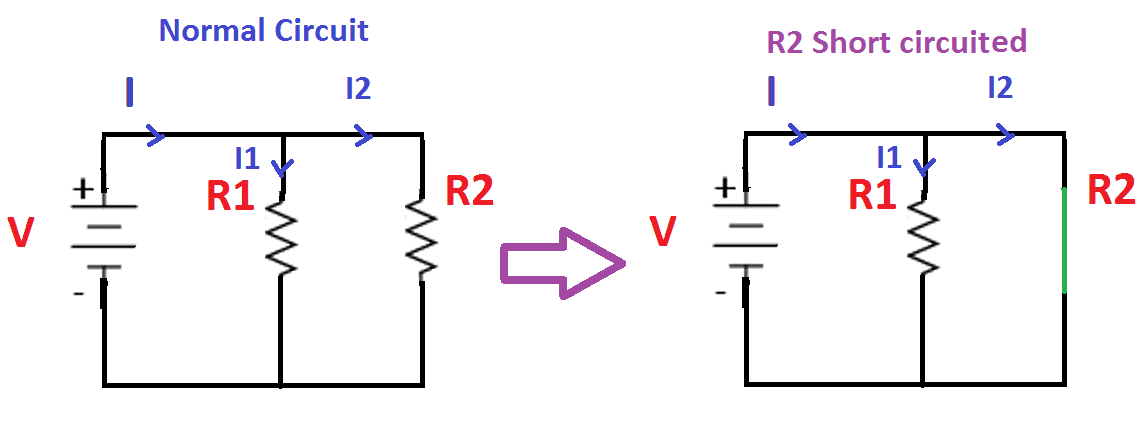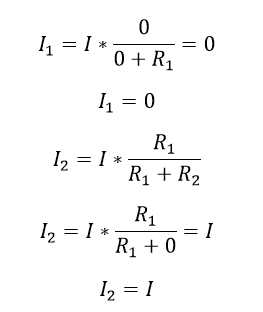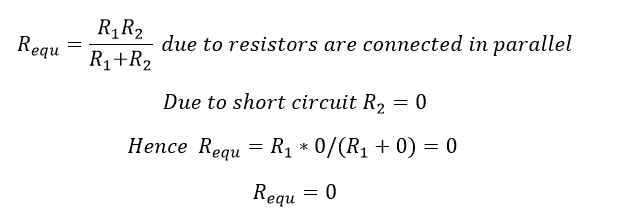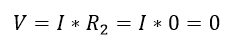# What is Short Circuit

Short circuits:

The circuit said to be short-circuited means where the component’s resistance is equal to zero.  Short circuit will happen due to unintended contact of components and consequent accidental diversion of the current. Short circuit is the challenging things in the electrical power system, to protect the equipment, we use special types of relays we see them later on. Let us see the basic idea about short circuit.Consider above mentioned drawing, V is the voltage across the battery, I is the total current from the battery to the load circuit, R1 is the load resistor (resistor) and R2 is another load resistor, both are connected in parallel with the battery and I1 and I2 is the current flowing through the resistor R1 and R2. Due to some accidental R2 resistor got shorted, the current through the resistor R2 is ….

Apply current divider rule…

Due to short circuit, the resistance is R2 resistor is zero. R2=0,Hence, the current flow through the resistance R1 is equal to zero…I1=0. Also the current flow through resistor R2 is equal to I…

Let see the equivalent resistance of the circuitHence Voltage across the short-circuited resistance..The best example for a short-circuit is, a switch operation:

A switch works as a short-circuit when the switch in ON condition, simultaneously the same switch works as an open circuit when the switch is an open condition.